# AP Statistics Curriculum 2007 NonParam 2MedianPair

## General Advance-Placement (AP) Statistics Curriculum - Differences of Medians of Two Paired Samples

Distribution-free (or non-parametric) statistical methods provide alternative to the (standard) parametric tests that we saw earlier, and are applicable when the distribution of the data is unknown.

## Motivational Clinical Example

Whitley and Ball reported on the relative risk of mortality from 16 studies of septic patients. The outcome measure of interest was whether the patients developed complications of acute renal failure. The relative risk calculated in each study compared the risk of dying between patients with and without renal failure. A relative risk of 1.0 means no effect, and relative risk $$\not= 1$$ suggests beneficial or detrimental effect of developing acute renal failure in sepsis. The main goal of the study was to determine whether developing acute renal failure as a complication of sepsis impacts patient mortality from the cumulative evidence in these 16 studies. The data of this study is included below.

 Study Relative Risk Sign (Relative Risk - 1) 1 0.75 - 2 2.03 + 3 2.29 + 4 2.11 + 5 0.80 - 6 1.50 + 7 0.79 - 8 1.01 + 9 1.23 + 10 1.48 + 11 2.45 + 12 1.02 + 13 1.03 + 14 1.30 + 15 1.54 + 16 1.27 +

We see the clear analogy of this study design to the Paired or One-Sample studies we saw before. However, if we were to plot these data (Relative Risk), we can see that their distribution is hardly symmetric, unimodal and bell-shaped (i.e., not Normal). Therefore, we cannot use the Paired T-test to test a Null-Hypothesis that the mean Relative Risk is 1 using this parametric test.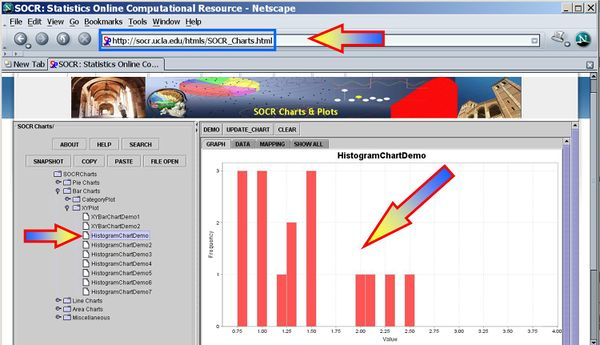## The Sign-Test

The Sign Test is a non-parametric alternative to the One-Sample and Paired T-Test. The Sign Test has no requirements for the data to be Normally distributed. It assigns a positive (+) or negative (-) sign to each observation according to whether it is greater or less than some hypothesized value. It measures the difference between the $$\pm$$ signs and how distinct the difference is from our expectations to observe by chance alone. For example, if there were no effects of developing acute renal failure on the outcome from sepsis, about half of the 16 studies above would be expected to have a relative risk less than 1.0 (a "-" sign) and the remaining 8 would be expected to have a relative risk greater than 1.0 (a "+" sign). In the actual data, 3 studies had "-" signs and the remaining 13 studies had "+" signs. Intuitively, this difference of 10 appears large to be simply due to random variation. If so, the effect of developing acute renal failure would be significant on the outcome from sepsis.

### Calculations

Suppose N+ is the number of "+" signs and we fix a significance level of $$\alpha= 0.05$$. And consider the following two hypotheses:

$H_o: N_+=8$ (equivalent to $$N_-=8$$): The effect of developing acute renal failure is not significant on the outcome from sepsis. $H_1: N_+ \not=8$: The effect of developing acute renal failure is significant on the outcome from sepsis.

Define the following test-statistics $B_s = \max{(N_+ , N_-)}$, where $$N_+$$ and $$N_-$$ are the number of positive and negative signs, respectively.

Then the distribution of $$B_s \sim Binomial(n=16, p=8/16=0.5)$$.

For our data, $$B_s = \max{(N_+ , N_-)}=\max{13,3}=13$$ and the probability that such binomial variable exceeds 13 is $$P(Bin(16,0.5,13))=0.010635$$. Therefore, we can reject the null hypothesis $$H_o$$ and regard as significant the effect of developing acute renal failure on the outcome from sepsis.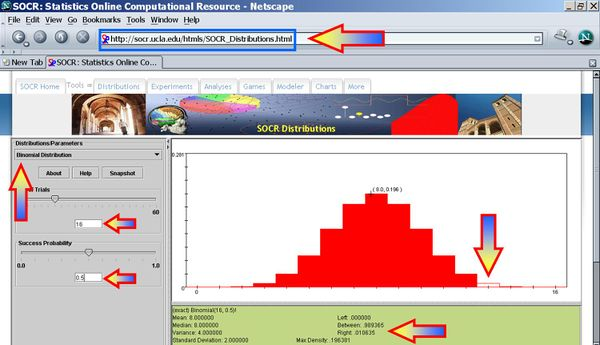### The Sign Test Using SOCR Analyses

It is much quicker to use SOCR Analyses to compute the statistical significance of the sign test. This SOCR Sign Test Activity may also be helpful in understanding how to use the sign test method in SOCR.

### Example

A set of 12 identical twins are given psychological tests to determine whether the first born of the set tends to be more aggressive than the second born. Each twin is scored according to aggressiveness; a higher score indicates greater aggressiveness. Because of the natural pairing in a set of twins these data can be considered paired.

 Twin-Index 1st Born 2nd Born Sign 1 86 88 - 2 71 77 - 3 77 76 + 4 68 64 + 5 91 96 - 6 72 72 0 (Drop) 7 77 65 + 8 91 90 + 9 70 65 + 10 71 80 - 11 88 81 + 12 87 72 +

We first plot the data using the SOCR Line Chart. Visually there does not seem to be a strong effect of the order of birth on baby's aggression.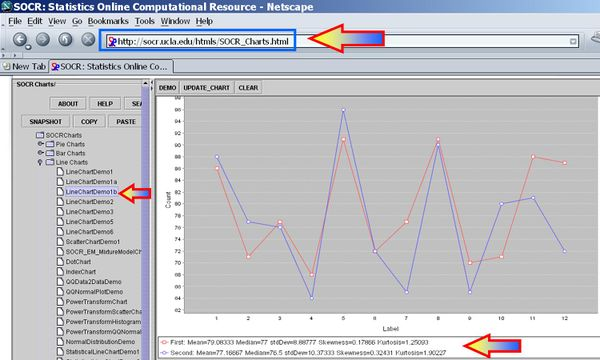Next, we can use the SOCR Sign Test Analysis to quantitatively evaluate the evidence to reject the null hypothesis that there is no birth-order effect on baby's aggressiveness.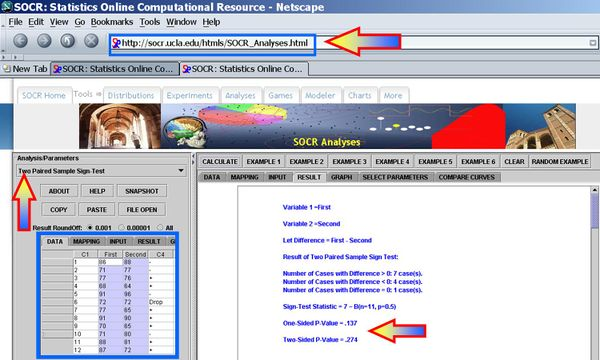Clearly the p-value reported is 0.274, and our data can not reject the null hypothesis.

## The Wilcoxon signed rank test

Like the sign test and the T-test, the Wilcoxon signed rank test involves comparisons of differences between measurements. It requires that the data are measured at an interval level of measurement, but does not require assumptions about the form of the distribution of the measurements. It should therefore be used whenever the distributional assumptions of the T-test are not satisfied.

### Example

Whitley and Ball reported data on the central venous oxygen saturation (SvO2 (%)) from 10 consecutive patients at 2 time points; at admission and 6 hours after admission to the intensive care unit (ICU). The null hypothesis is that there is no effect of 6 hours of ICU treatment on SvO2. Under the null hypothesis, the mean of the differences between SvO2 at admission and that at 6 hours after admission should be zero.

 Patient On Admission At 6 Hours Difference Rank 2 59.1 56.7 -2.4 1 7 58.2 60.7 2.5 2 9 56.0 59.5 3.5 3 10 65.3 59.8 -5.5 4 3 56.1 61.9 5.8 5 5 60.6 67.7 7.1 6 6 37.8 50.0 12.2 7 1 39.7 52.9 13.2 8 4 57.7 71.4 13.7 9 8 33.6 51.3 17.7 10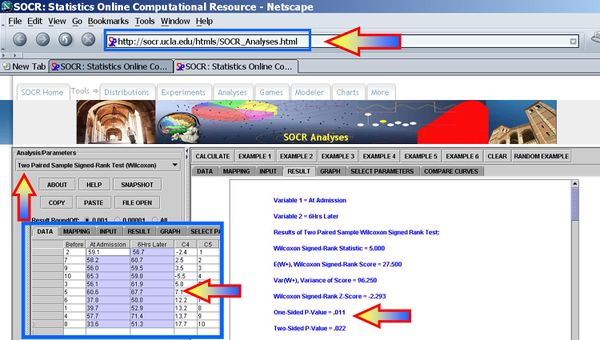Clearly, we can reject the null-hypothesys at $$\alpha=0.05$$, as the one- and two-sided alternative hypotheses p-values for the Wilcoxon signed rank test reported by the SOCR Analysis are respectively

One-Sided p-value = 0.011
Two-Sided p-value = 0.022

## Practice Problems

Suppose 10 randomly selected rats were chosen to see if they could be trained to escape a maze. The rats were released and timed (sec.) before and after 2 weeks of training (N means the rat did not complete the maze-test). Do the data provide evidence to suggest that the escape time of rats is different after 2 weeks of training? Test using $$\alpha= 0.05$$.

 Rat Before After Sign 1 100 50 + 2 38 12 + 3 N 45 + 4 122 62 + 5 95 90 + 6 116 100 + 7 56 75 - 8 135 52 + 9 104 44 + 10 N 50 +